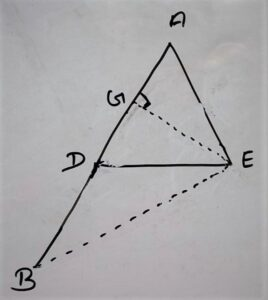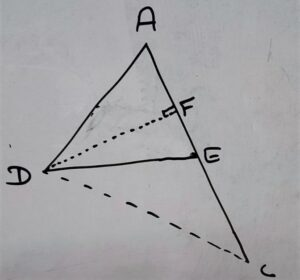# State and Prove Basic Proportionality (BPT) Theorem Class 10

Theorem 6.1 Thale’s Theorem/ Basic Proportionality Theorem (BPT)

Statement: ” If a line is drawn parallel to one side of a triangle to intersect the other two sides in distinct points. The other two sides are divided in the same ratio.”

OR

Prove that in a triangle a line parallel to one side divides remaining two sides in the same ratio

Given:

In Δ ABCDE // ( parallel to ) BC

To Prove:

Construction:

DF ⊥ ( Perpendicular ) on AC

EG ⊥ ( Perpendicular ) on AB

Join B to E and  C to D

Proof:

According to figure:

In Δ ADE and Δ BDEhave common altitude (Perpendicular) = EG

∴ area Δ ADE /area Δ BDE = 1/2×b×h / 1/2×b×h

area Δ ADE /area Δ BDE = AD / BD ————-equation (i)

Similarly

In Δ ADE and Δ CEDHave common altitude(perpendicular) = DF

ar Δ ADE / ar Δ CED = 1/2×b×h / 1/2×b×h

⇒ ar Δ ADE / ar Δ CED = AE×DF / CE×DF

⇒ ar Δ ADE / ar Δ CED = AE / CE ————-equation (ii)

Δ BDE and Δ CED are lies on Common base (DE) and between same parallel lines

∴ ar Δ BDE = ar Δ CED

Dividing both side by area Δ ADE

⇒ ar Δ BDE/ar Δ ADE = ar Δ CED/ar Δ ADE

⇒ ar Δ ADE/ar Δ BDE = ar Δ ADE/ar Δ CED [ After reciprocal of both side] ————–equation(iii)

By comparing equation (i) and equation(ii) with equation (iii) We get